# Euler method

A very simple finite-difference method for the numerical solution of an ordinary differential equation. Let a differential equation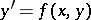(1)

with initial condition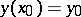be given. A sufficiently small stepon the-axis is chosen and points,are constructed. One then replaces the desired integral curve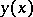by a polygonal line (Euler's polygonal line) whose segments are rectilinear on the intervals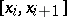; and the ordinates of the end points of these segments are determined from the formulas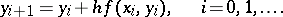(2)

If the right-hand side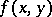of (1) is continuous, then the sequence of Euler polygonal lines converges uniformly, as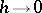, to the unknown curveon a sufficiently small interval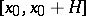.

The Euler method consists in representing the integral of the differential equation (1) on every intervalby the first two terms of its Taylor series: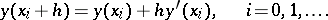The error of this method is of order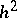for every step.

The Euler method can be refined by means of various modifications. For example, an improved method of polygonal lines is obtained by replacing the formula (2) for the computation of the ordinates by the relation(3)

where(4)

that is, by taking into account the direction of the field of integral curves at the midpoints (4) of the segments of the polygonal line.

Another modification leads to the improved Euler–Cauchy method: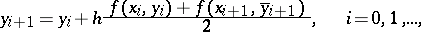(5)

whereThe last method can be refined even further by using an iterative scheme to improve the value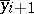:(6)

where the zero-approximation is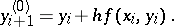The iterative computation by means of (6) is continued until two consecutive approximations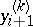and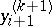coincide in a prescribed number of decimal places. If after three or four iterations this does not happen, the stepshould be made smaller. The error of the Euler method with iterative computation of the ordinates is of order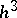for every step.

Euler's method and its modifications carry over to the more general case of solving a system ofordinary differential equationswith given initial conditions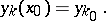The numerical algorithm of the Euler method can easily be programmed on a computer.

This method was proposed by L. Euler (1768).